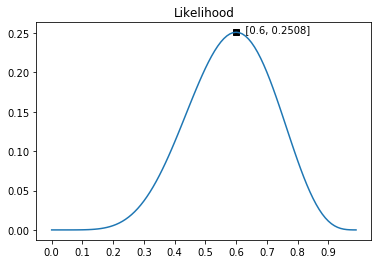# 最大似然估计：从概率角度理解线性回归的优化目标

## 概率和似然

### 概率

$P(6正4反 \ |\ \theta=0.5)=C_{10}^{6}\times 0.5^6 \times (1-0.5)^4 \approx 0.2051 \\ P(6正4反 \ |\ \theta=0.6)=C_{10}^{6}\times 0.6^6 \times (1-0.6)^4 \approx 0.2508 \\ P(6正4反 \ |\ \theta=0.9)=C_{10}^{6}\times 0.9^6 \times (1-0.9)^4 \approx 0.0112$

### 似然

$L(\theta ; 6正4反)=C_{10}^{6}\times \theta^6 \times (1-\theta)^4 \\$“6正4反”的似然函数

## 最大似然估计

### 线性回归的最大似然估计

$P(\epsilon^{(i)}) = \frac{1}{\sqrt{2 \pi \sigma^2}}\exp{({-\frac{(\epsilon^{(i)})^2}{2 \sigma^2}})}$

$\log (ab) = \log(a) + \log(b) \\ \log_e (\exp(a)) = a$

1. 对目标函数求导，让导数为0，得到一个矩阵方程，矩阵方程的解即为模型的最优解
2. 使用梯度下降法，求模型的最优解。

## 总结

1. 如何通俗地理解概率论中的「极大似然估计法」? - 马同学的回答 - 知乎 https://www.zhihu.com/question/24124998/answer/242682386
2. Andrew Ng：CS229 Lecture Notes